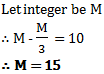# Test: Integers - 1

## 10 Questions MCQ Test Mathematics (Maths) Class 7 | Test: Integers - 1

Description
This mock test of Test: Integers - 1 for Class 7 helps you for every Class 7 entrance exam. This contains 10 Multiple Choice Questions for Class 7 Test: Integers - 1 (mcq) to study with solutions a complete question bank. The solved questions answers in this Test: Integers - 1 quiz give you a good mix of easy questions and tough questions. Class 7 students definitely take this Test: Integers - 1 exercise for a better result in the exam. You can find other Test: Integers - 1 extra questions, long questions & short questions for Class 7 on EduRev as well by searching above.
QUESTION: 1

### Which integer is greater than –5?

Solution:

An integer on the horizontal number line is greater than the number on its left and less than the number on its right. Therefore, smallest negative integer is biggest one and bigger integer will be smaller ones

So -1 will be greater than -5.

So option B is the correct answer.

QUESTION: 2

### If a x (b −c) is 8 for a = 2, b = 10 and c = 6 then a x b − a x c is equal to

Solution:

Using BODMAS

a x (b −c) = a x b - a x c

= 2 x 10 - 2 x 6
= 20 - 12
= 8

So, option D is the correct answer.

QUESTION: 3

### What is the absolute value of |-45|?

Solution:

The absolute value of any number is the magnitude of the real number without regard to its sign. The magnitude of a number is its size.

This means |-45|=45

QUESTION: 4

What is the absolute value of |-144|?

Solution:

The absolute value of any number is the magnitude of the real number without regard to its sign. The magnitude of a number is its size.

This means |-144|=144

Therefore, Option A is the correct answer.

QUESTION: 5

According to Distributive Law of Multiplication over Addition, the a x (b + c) must be equal to

Solution:

Acoording to Distributive Law of Multiplication over Addition :

► a × (x + y) = ax + ay

According to question ,a x (b + c) = a x b + a x c

QUESTION: 6

Which amongst the following is the largest?

I-89I, -89, -21, I-21I

Solution:

I-89I = 89

I-21I = 21

So, 89 is the greatest amongst the given numbers.

QUESTION: 7

What is the absolute value of |-2677|?

Solution:

Absolute value describes the distance of a number on the number line from 0 without considering which direction from zero the number lies. The absolute value of a number is never negative.

So absolute value of |-2677| is 2677

So option A is the correct answer.

QUESTION: 8

What is the absolute value of |-239|?

Solution:

The absolute value of a number is how far away the number is from zero. The absolute value is ALWAYS positive.

So absolute value of |-239| = 239

So option B is the correct answer.

QUESTION: 9

How many integers are there between –8 and 2 (excluding -8 and 2)?

Solution:

The integers between -8 & 2 are : -7 , -6 , -5 , -4 , -3 , -2 , -1 , 0 , 1

So there are 9 integers between -8 & 2

So , option D is correct.

QUESTION: 10

An integer is 10 more than its one-third part. The integer is

Solution:So option A is the correct answer.